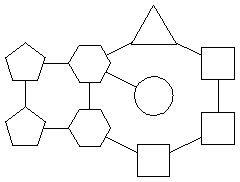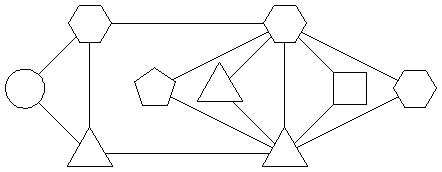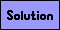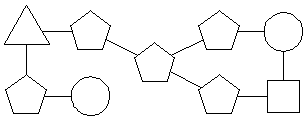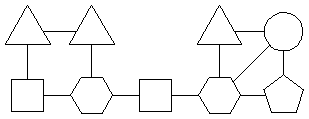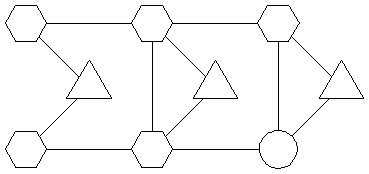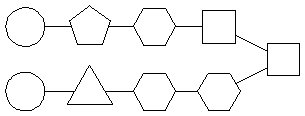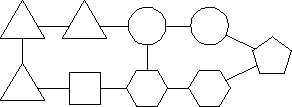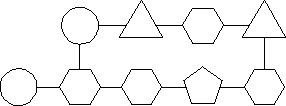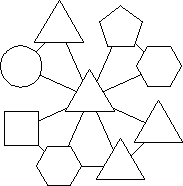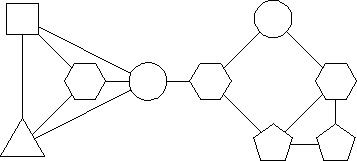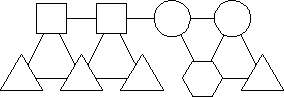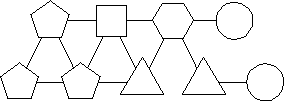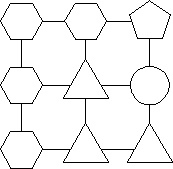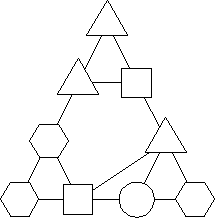Each digit, 1 through 9, should be placed into the shapes below. Each digit in a triangle should be the first digit of the product of neighboring digits. Each digit in a square should be the last digit of the product of neighboring digits. Each digit in a pentagon should be the first digit of the sum of neighboring digits. Each digit in a hexagon should be the last digit of the sum of neighboring digits. There are no restrictions on numbers contained in circles.

Each puzzle below has a unique solution.